## Fluid Mechanics and Hydraulic Machinery Miscellaneous

#### Fluid Mechanics and Hydraulic Machinery

1. A U-tube manometer with a small quantity of mercury is used to measure the static pressure difference between two locations A and B in a conical section through which an in compressible fluid flows. At a particular flow rate, the mercury column appears as shown in the figure. The density of mercury is 13600 kg/ m3 and g - 9.81 m/s2. Which of the following is correct?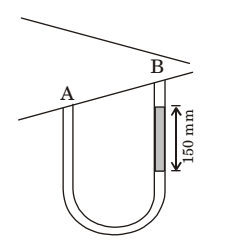1.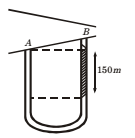PA – PB = rg. Dh
= 13600 × 9.81 × .15
= 20 kPa
As pressure is decreasing from A to B, so flow direction is A to B.

##### Correct Option: APA – PB = rg. Dh
= 13600 × 9.81 × .15
= 20 kPa
As pressure is decreasing from A to B, so flow direction is A to B.

1. A venturimeter of 20 mm throat diameter is used to measure the velocity of water in a horizontal pipe of 40 mm diameter. If the pressure difference between the pipe and throat sections is found to be 30 kPa then, neglecting frictional losses, the flow velocity is

1. We know, A1 V1 = A2 V2

 ƥ V2 = D21 V1 = 16 V1 D22 4

∴ V2 = 4V1
Applying Bernoulli's Equation
 p1 + v21 + z1 = p2 + p22 + z2 ρg 2g ρg 2g

 P1 - P2 = V22 - V21 eg 2g

 ƥ 15V21 = 30 × 103 2 1000

ƥ V21 = 4
ƥ V1 = 2.0 m/s
So velocity of flow is 2.0 m/sec

##### Correct Option: D

We know, A1 V1 = A2 V2

 ƥ V2 = D21 V1 = 16 V1 D22 4

∴ V2 = 4V1
Applying Bernoulli's Equation
 p1 + v21 + z1 = p2 + p22 + z2 ρg 2g ρg 2g

 P1 - P2 = V22 - V21 eg 2g

 ƥ 15V21 = 30 × 103 2 1000

ƥ V21 = 4
ƥ V1 = 2.0 m/s
So velocity of flow is 2.0 m/sec

1. Air flows through a venturi and into atmosphere. Air density is ρa; atmospheric pressure is ρa; throat diameter is Dt; exit diameter is D and exit velocity is U. The throat is connected to a cylinder containing a friction less piston attached to a spring. The spring constant is k. The bottom surface of the piston is exposed to atmosphere. Due to the flow, the piston moves by distance x. Assuming in compressible friction less flow, x is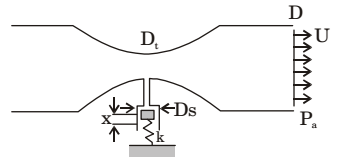1.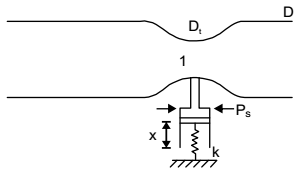Applying Bernoulli’s equation at points (1) and (2), we have

 p1 + v21 + z1 = p2 + p22 + z2 ρg 2g ρg 2g

Since venturi is horizontal
z1 = z2
 Now p1 - p2 = v22 - p21 ρg ρg 2g 2g

 ⇒(p1 - p2) = ρg (v22 - v21) = ρ = (v22 - v21) 2g g

Since P2 = Pa = atmospheric pressure
 ∴ (P2 - Pa) ρ (v22 - v21) ........(i) 2

Applying continuity equation at points (i) and (ii), we have
A1 v1 = A2 v2
 ⇒ v1 =A2v2 since V2 = U A1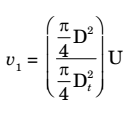v1 =D2 U Dt

From equation (i),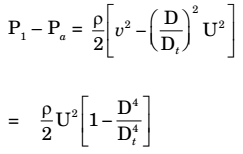At point P
Spring force = pressure force due air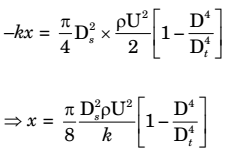##### Correct Option: DApplying Bernoulli’s equation at points (1) and (2), we have

 p1 + v21 + z1 = p2 + p22 + z2 ρg 2g ρg 2g

Since venturi is horizontal
z1 = z2
 Now p1 - p2 = v22 - p21 ρg ρg 2g 2g

 ⇒(p1 - p2) = ρg (v22 - v21) = ρ = (v22 - v21) 2g g

Since P2 = Pa = atmospheric pressure
 ∴ (P2 - Pa) ρ (v22 - v21) ........(i) 2

Applying continuity equation at points (i) and (ii), we have
A1 v1 = A2 v2
 ⇒ v1 =A2v2 since V2 = U A1v1 =D2 U Dt

From equation (i),At point P
Spring force = pressure force due air1. Water flows though a vertical contraction from a pipe of diameter d to another of diameter d/2 (see fig.) The flow velocity at the inlet to the contraction is 2 m/s and pressure 200 kN/m2. If the height of the contraction measures 2m,then pressure at the exit of the contraction will be very nearly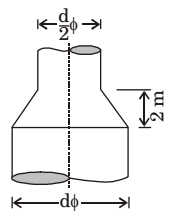1. From continuity equation,
A1 v1 = A2 v2

 or π × d2 × 2 = πd2 × v2 4 4 2

or v2 = 8 m/s.
Applying Bernoulli's theorem,
 p1 + v21 + z1 = p2 + p22 + z2 w 2g w 2g

 or 200 × 1000 + (2)2 + 0 9810 2 × 9.81

 or p2 + (8)2 + 2 w 2 × 9.81

or p2 =150.38 kN/m2

##### Correct Option: C

From continuity equation,
A1 v1 = A2 v2

 or π × d2 × 2 = πd2 × v2 4 4 2

or v2 = 8 m/s.
Applying Bernoulli's theorem,
 p1 + v21 + z1 = p2 + p22 + z2 w 2g w 2g

 or 200 × 1000 + (2)2 + 0 9810 2 × 9.81

 or p2 + (8)2 + 2 w 2 × 9.81

or p2 =150.38 kN/m2

1. In a venturimeter, the angle of the diverging section is more than that of converging section. State: (T/F)

1. False

False